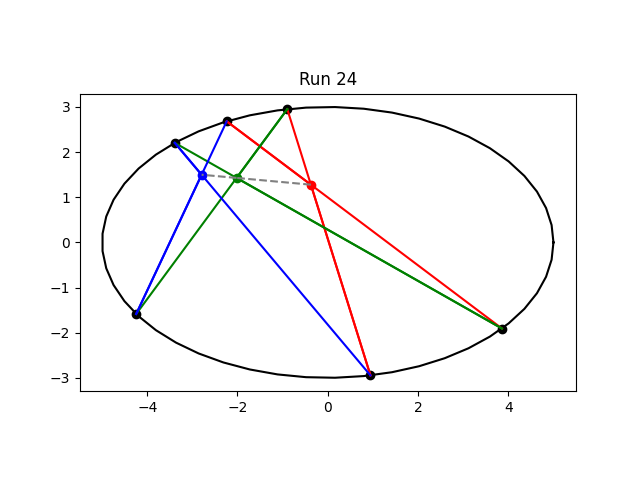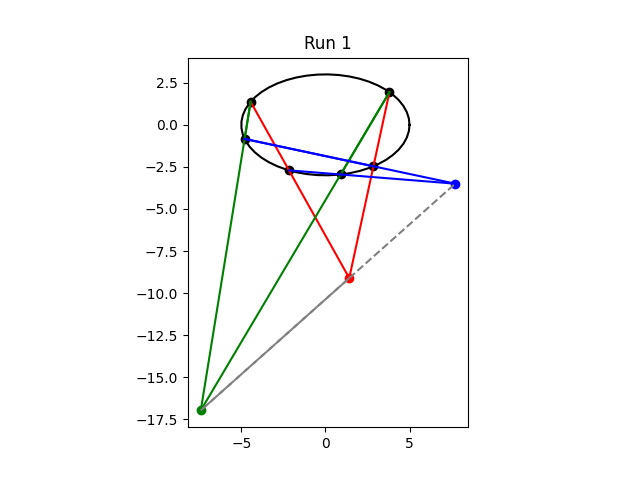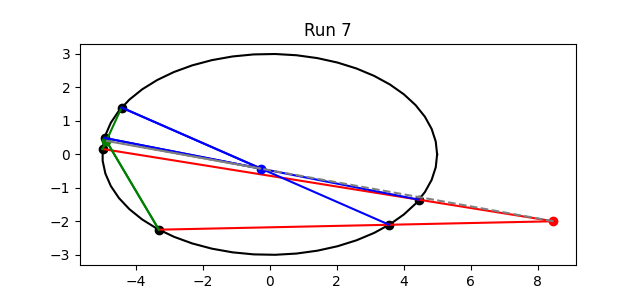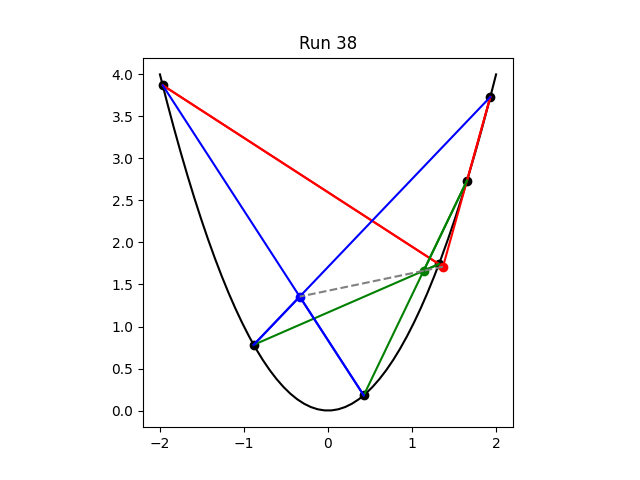# Random illustrations of Pascal’s theorem

Pascal’s theorem begins by selecting any six distinct points on an ellipse and drawing a “hexagon.” I put hexagon in quotes because the result need not look anything like a hex nut. In this context it simply means to pick one point, connect it to some other point, and so forth, joining the points in a cycle. The points need not be in order around the ellipse, and so the hexagon can, and usually will, be irregularly shaped.

Pascal’s theorem says that the intersections of opposite sides intersect in three points that lie in on a common line. Since the hexagon can be irregularly shaped, it’s not immediately clear what the “opposite” sides are.

The segment joining points 1 and 2 is opposite the segment joining points 4 and 5. The segment joining points 2 and 3 is opposite the segment joining points 5 and 6.The segment joining points 3 and 4 is opposite the segment joining points 6 and 1. If the hexagon were a regular hexagon, opposite sides would be parallel.

The theorem is usually illustrated with something like the figure below.Opposite sides share the same color. The blue, green, and red dots inside the ellipse are the intersections of the blue sides, the green sides, and the red sides respectively. And as Pascal predicted, the three points are colinear.

The statement of Pascal’s theorem above is incomplete. We have to look at where the lines containing the sides intersect, not where the sides intersect. In the image above, the lines intersect where the line segments intersect, but this doesn’t always happen.

The Wikipedia article on Pascal’s theorem says “the three pairs of opposite sides of the hexagon (extended if necessary) meet at three points which lie on a straight line.” The parenthetical remark to extend the sides “if necessary” could lead you to think that needing to extend the sides is the exceptional case. It’s not; it’s the typical case.

The graph above is typical of presentations of Pascal’s theorem, but not typical of randomly selected points on an ellipse. I wrote a program to select random points on an ellipse and connect them as the theorem requires. The title of the plot above is “Run 24” because that was the first run to produce a typical illustration.

Here’s what the first run looked like.In this example, all three intersection points are outside the ellipse. It’s hard to see the blue side on the bottom of the ellipse; it’s so short that the ellipse is practically flat over that distance and the blue line lies nearly on top of the black arc of the ellipse. This is a more typical example: usually one or more intersection points lies outside the ellipse, and usually one or more sides is hard to see.

Here’s an example that is even more typical.Seeing this kind of plot first make me think there was a bug in my program. The shorter green side is hard to see, the intersection of the two green lines is lost between two points clumped close together, and the gray line connecting the three intersection points lies practically on top of a couple other lines.

## Random examples and random tests

Random examples are complementary to clean classroom examples. The first image, Run 24, is typical in presentations for good reasons: it’s easy to see all the sides, and all the action happens in a small area. But random examples give you a better appreciation for the range of possibilities. This is very similar to software testing: some tests should be carefully designed and some should be chosen at random.

## Conic sections

Pascal’s theorem applies to conic sections in general, not just ellipses. Here is an example with a parabola.Randomly selected examples for parabolas are even less legible than random examples for ellipses. It’s common for lines to pile up on top of each other.

## Projective geometry

Strictly speaking Pascal’s theorem is a theorem in projective geometry. It tacitly assumes that the lines in question do intersect. In the Euclidean plane two of the sides might be parallel and not intersect. In projective geometry parallel lines intersect “at infinity.” Or rather, there are no parallel lines in projective geometry.

With randomly selected points, the probability of two lines being parallel is 0. But it is possible that two lines are nearly parallel and intersect far from the ellipse. Some of the runs I made for this post did just this, like Run 9 below.If you’ve got room for one illustration, you’d naturally use something like Run 24, but it’s good to know that things like Run 9 can happen too.